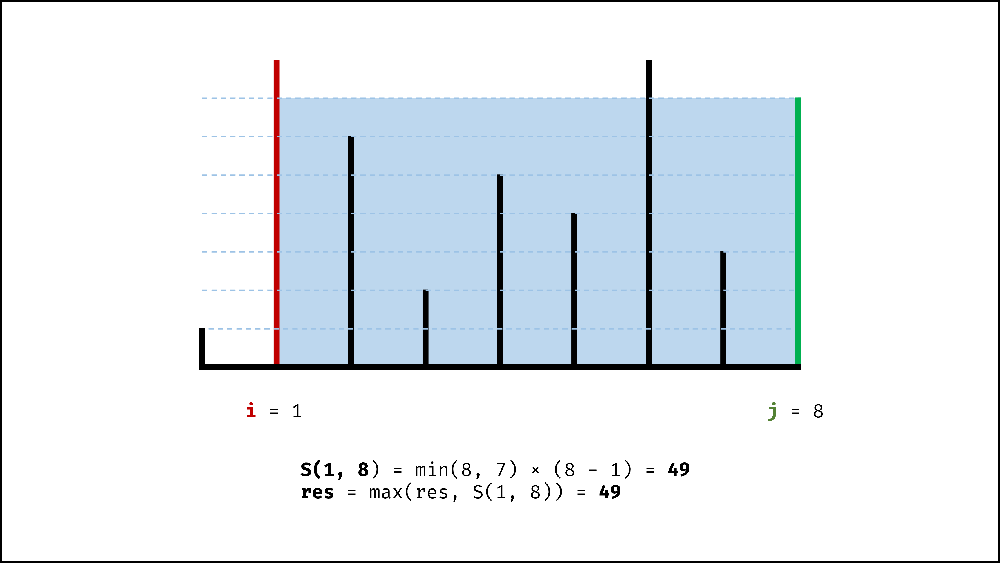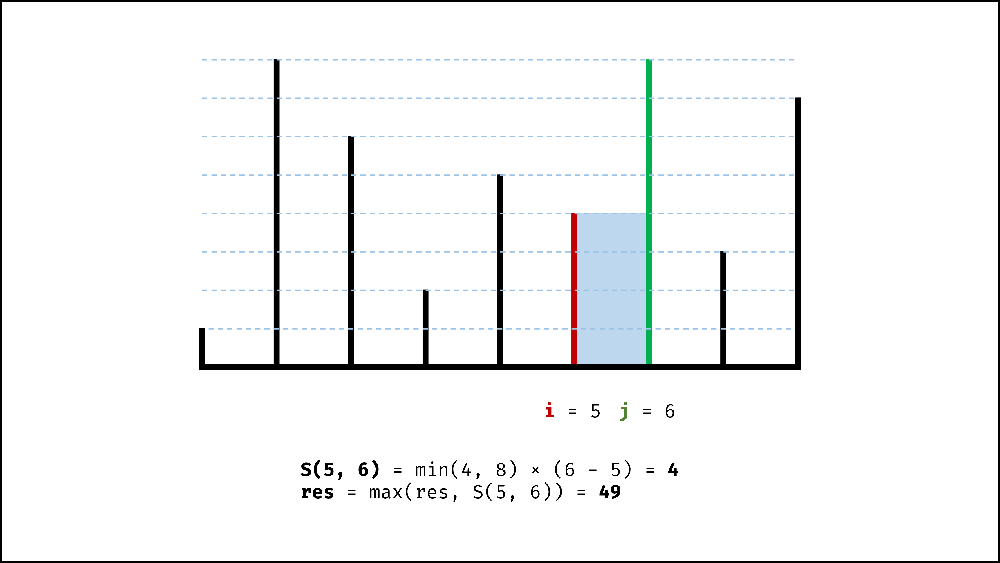By Long Luo

Here shows 2 Approaches to slove this problem: Brute Force and Two Pointers.

# IntuitionSuppose two pointers $i$, $j$, the heights of the vertical lines are $h[i]$, $h[j]$, and the area in this state is $S(i, j)$.

As we all known, the container is determined by the short line, the area formula can be easily obtained:

$S(i, j)= min(h[i], h[j]) \times (j - i)$

# Brute Froce

It’s easy to use the brute force approach, the total states is $C(n, 2)= n \times (n - 1) / 2$, we have to enumerate all these states to get the max area.

The time complexity is $O(n^2)$, exceed the time limit.

## Analysis

• Time Complexity: $O(n^2)$
• Space Complexity: $O(1)$

# Two Pointers

In each state $S(i, j)$, no matter whether the left line or right line moves to the middle, it will cause less wide to $width - 1$:

• Move the short line, the short line $min(h[i], h[j])$ of the container may hold more water, the area may increase.
• Move the long line, the short line $min(h[i], h[j])$ of the container will remain the same or less, so the area will definitely become less.

Therefore, we can use two pointers to the left and right line of the container. We move the short line in each round, update the max area until the two pointers met each other as the below pictures show.## Proof

Assuming that $h[i] \lt h[j]$ under the state $S(i, j)$, move the short line to $S(i + 1, j)$, which means that we eliminate $S(i, j - 1), S(i, j - 2), ... , S(i, i + 1)$ states. The area of all eliminated states must be smaller than the current area:

1. Short line height: same or less than $S(i, j)$ ($\le h[i]$);
2. Width: less than $S(i, j)$.

Therefore, each round moves the short line, and all the eliminated states will not cause the loss of the maximum area.

## Analysis

• Time Complexity: $O(n)$
• Space Complexity: $O(1)$

All suggestions are welcome.
If you have any query or suggestion please comment below.
Please upvote👍 if you like💗 it. Thank you:-)

Explore More Leetcode Solutions. 😉😃💗